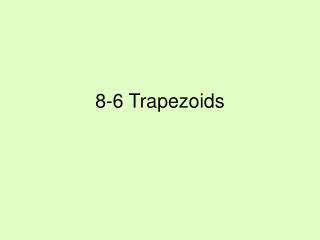DownloadDownload Presentation8-6 Trapezoids

# 8-6 Trapezoids

Télécharger la présentation## 8-6 Trapezoids

- - - - - - - - - - - - - - - - - - - - - - - - - - - E N D - - - - - - - - - - - - - - - - - - - - - - - - - - -
##### Presentation Transcript

1. 8-6 Trapezoids

2. Properties of Trapezoids • Quadrilateral with one pair of parallel sides • The bases are the parallel sides • The non parallel sides are legs • If the legs are congruent then the trapezoid is isosceles • Both pairs of base angles of an isosceles trapezoid are congruent • The diagonals of an isosceles trapezoid are congruent

3. Isosceles Trapezoid A B D C

4. Medians • The segment that joins the midpoints of the legs of a trapezoid • The median of a trapezoid is parallel to the bases • The measure of the median is one-half the sum of the measures of the bases A B E F D C

5. Example: • DEFG is an isosceles trapezoid with median MN • Find DG if EF=20 and MN=30 • Find m<1, m<2, m<3 and m<4 if m<1=3x+5 and m<3=6x-5 E F 3 4 M N 1 2 D G

6. G F Proof C D • CDFG is an isosceles trapezoid with bases CD and FG. Prove that